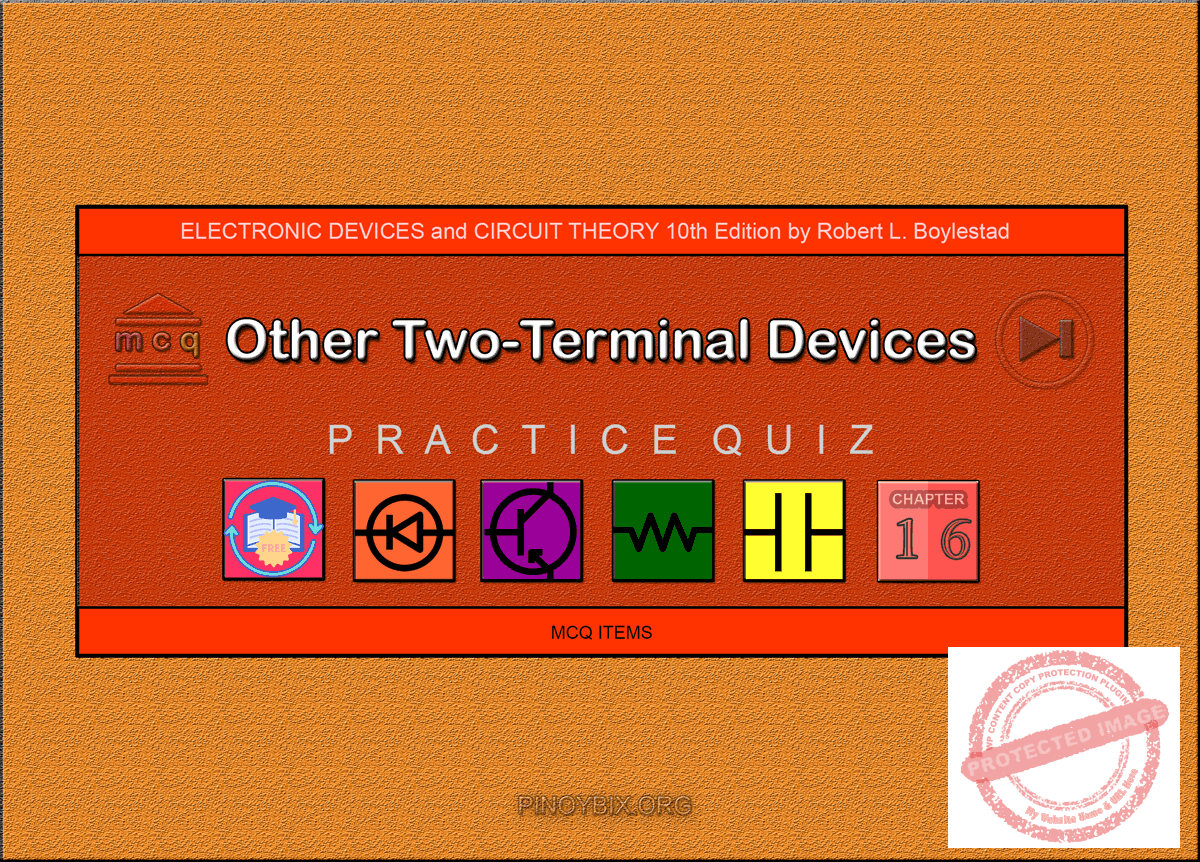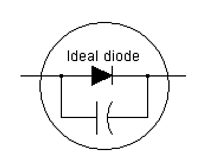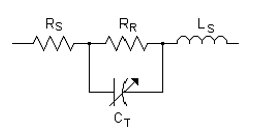# Boylestad: MCQ in Other Two-Terminal Devices

(Last Updated On: December 2, 2019)This is the Multiple Choice Questions in Other Two-Terminal Devices from the book Electronic Devices and Circuit Theory 10th Edition by Robert L. Boylestad. If you are looking for a reviewer in Electronics Engineering this will definitely help. I can assure you that this will be a great help in reviewing the book in preparation for your Board Exam. Make sure to familiarize each and every questions to increase the chance of passing the ECE Board Exam.

#### Online Questions and Answers Topic Outline

• MCQ in Introduction to Other Two-Terminal Devices | MCQ in Schottky Barrier (Hot-Carrier) Diodes | MCQ in Varactor (Varicap0 Diodes | MCQ in Power Diodes | MCQ in Tunnel Diodes | MCQ in Photodiodes | MCQ in Photoconductive Cells | MCQ in IR Emitters | MCQ in Liquid-Crystal Displays (LCD) | MCQ in Solar Cells | MCQ in Thermistors

#### Practice Exam Test Questions

Choose the letter of the best answer in each questions.

1. Which of the following is (are) diodes?

A. Schottky

B. Varactor

C. Tunnel

D. All of the above

Solution:

2. Which of the following metals is (are) used in the fabrication of Schottky diodes?

A. Molybdenum

B. Platinum

C. Tungsten

D. All of the above

Solution:

3. What are the typical ranges of reverse-bias current levels IS for low-power and high-power Schottky diodes at room temperature?

A. Picoamperes, nanoamperes

B. Nanoamperes, microamperes

C. Microamperes, milliamperes

D. Milliamperes, amperes

Solution:

4. What is the voltage drop across Schottky diodes?

A. 0 V to 0.2 V

B. 0.7 V to 0.8 V

C. 0.8 V to 1.0 V

D. 1.0 V to 1.5 V

Solution:

5. What metal(s) is(are) used in the construction of Schottky diodes?

A. Molybdenum

B. Platinum

C. Tungsten

D. Silicon

E. Any of the above

Solution:

6. For a 50-A unit, the PIV of the Schottky is about _____ compared to 150 V for the p-n junction variety.

A. 25

B 50

C. 75

D. 100

Solution:

7. Schottky diodes are very effective at frequencies approaching ______.

A. 20 GHz

B. 10 MHz

C. 100 MHz

D. 1 MHz

Solution:

8. This is an approximate equivalent circuit for the ______ diode.A. Schottky

B. varicap

C. tunnel

Solution:

9. What is the range of the varying capacitor CT in varactor diodes?

A. 0 pF to 5 pF

B. 2 pF to 100 µF

C. 2 µF to 100 µF

D. 2 pF to 100 pF

Solution:

10. Which of the following areas is (are) applications of varactor diodes?

A. FM modulators

B. Automatic-frequency control devices

D. All of the above

11. The tuning diode is a ______-dependent, variable ______.

A. voltage, resistor

B. current, capacitor

C. voltage, capacitor

D. current, inductor

Solution:

12. This is an equivalent circuit for the ______ diode.A. Schottky

B. varicap

C. tunnel

D. any of the above

Solution:

13. The varicap diode has a transition capacitance sensitive to the applied reverse-bias potential that is a maximum at zero volts and decreases ______ with increasing reverse-bias potentials.

A. logarithmically

B. parabolically

C. exponentially

D. all of the above

Solution:

14. The majority of power diodes are constructed using ______.

A. molybdenum

B. platinum

C. tungsten

D. silicon

Solution:

15. The current capability of power diodes can be increased by placing two or more in series.

A. True

B. False

Solution:

16. The PIV rating of power diodes can be increased by stacking the diodes in series.

A. True

B. False

Solution:

17. Which of the following diodes has a negative-resistance region?

A. Schottky

B. Varactor

C. Tunnel

D. Power

Solution:

18. Which of the following semiconductor materials is (are) used in the manufacturing of tunnel diodes?

A. Germanium

B. Gallium

C. Both germanium and gallium arsenide

D. Silicon

Solution:

19. What is the ratio IP / IV for gallium arsenide?

A. 1:1

B. 5:1

C. 10:1

D. 20:1

Solution:

20. What is the limit of peak current IP in tunnel diodes?

A. A few microamperes to several hundred amperes

B. A few microamperes to several amperes

C. A few microamperes to several milliamperes

D. A few microamperes to several hundred microamperes

Solution:

21. What is the maximum peak voltage for tunnel diodes?

A. 50 mV

B. 100 mV

C. 250 mV

D. 600 mV

Solution:

22. In which region is the operating point stable in tunnel diodes?

A. Negative-resistance

B. Positive-resistance

C. Both negative- and positive-resistance

D. Neither negative- nor positive-resistance

Solution:

23. Which of the following diodes is limited to the reverse-bias region in its region of operation?

A. Schottky

B. Tunnel

C. Photodiode

D. Rectifier

Solution:

24. What is the response time of cadmium sulfide (CdS) in photoconductive cells?

A. 100 ms

B. 50 ms

C. 25 ms

D. 10 ms

Solution:

25. Which of the following areas is (are) an application of infrared-emitting diodes?

A. Intrusion alarms

B. Shaft encoders

D. All of the above

Solution:

26. What is the maximum temperature limit for liquid-crystal displays (LCDs)?

A. 10°C

B. 30°C

C. 60°C

D. 100°C

Solution:

27. What is the response time of light-emitting diodes (LEDs)?

A. Less than 100 ns

B. 50 ms

C. 100 ms to 300 ms

D. 400 ms

Solution:

28. What is the response time of LCDs?

A. Less than 100 ns

B. 50 ms

C. 100 ms to 300 ms

D. 400 ms

Solution:

29. What is the power density received from the sun at sea level?

A. 10 mW/cm2

B. 100 mW/cm2

C. 500 mW/cm2

D. 1 W/cm2

Solution:

30. Which of the following semiconductor materials is (are) used for manufacturing solar cells?

A. Gallium arsenide

B. Indium arsenide

D. All of the above

Solution:

31. What type of temperature coefficient do thermistors have?

A. Positive

B. Negative

C. Either positive or negative

D. None of the above

Solution:

32. Which of the following materials is (are) used in the manufacturing of thermistors?

A. Ge

B. Si

C. A mixture of oxides of cobalt, nickel, strontium, or manganese

D. All of the above

Solution:

33. What is the resistance of thermistors at room temperature (20°C)?

A. 5 kΩ

B. 1 kΩ

C. 100 Ω

D. 1 Ω

Solution:

34. What is the resistance of thermistors at boiling temperature (100°C)?

A. 5 kΩ

B. 1 kΩ

C. 100 Ω

D. 1 Ω

Solution:

35. What is the typical level of change in resistance per degree change in temperature?

A. 1% to 2%

B. 3% to 5%

C. 7% to 10%

D. 10% to 25%

Solution:

#### FILL-IN-THE-BLANKS

1. Schottky diodes have ______.

A. quick response time

B. a lower noise figure

C. both quick response time and a lower noise figure

D. None of the above

Solution:

2. Schottky diode construction results in a ______ uniform junction region and a ______ level of ruggedness.

A. more, high

B. less, high

C. more, low

D. less, low

Solution:

3. In both n-type and p-type silicon materials, the ______ is the majority carrier in a Schottky diode.

A. hole

B. electron

C. proton

C. neutron

Solution:

4. The barrier at the junction for a Schottky diode is ______ that of the p-n junction device in both the forward- and reverse-bias regions.

A. the same as

B. more than

C. less than

D. None of the above

Solution:

5. A Schottky diode has ______ level of current at the same applied bias compared to that of the p-n junction at both the forward- and reverse-bias regions.

A. a lower

B. a higher

C. the same

D. None of the above

Solution:

6. The PIV of Schottky diodes is usually ______ that of a comparable p-n junction unit.

A. 1/2

B. 1/3

C. 1/4

D. 1/5

Solution:

7. Varactor diodes are ______.

A. semiconductor devices

B. voltage-dependent

C. variable capacitors

D. All of the above

Solution:

8. In varactor diodes, as the reverse-bias potential increases, the width of the depletion region ______, which in turn ______ the transition capacitance.

A. increases, increases

B. decreases, reduces

C. increases, reduces

D. decreases, increases

Solution:

9. The normal range of reverse-bias voltage VR for varactor diodes is limited to about ______.

A. 15 V

B. 20 V

C. 25 V

D. 40 V

Solution:

10. In the reverse-bias region of varactor diodes, the resistance RR in parallel with the varying capacitor is ______ and the series resistance RS is ______.

A. very large, very small

B. very large, very large

C. very small, very large

D. very small, very small

Solution:

11. The majority of power diodes are constructed using silicon because of its higher ______ rating(s).

A. current

B. temperature

C. PIV

D. All of the above

Solution:

12. The current capability of power diodes can be increased by placing two or more of the diodes in ______, and the PIV rating can be increased by stacking the diodes in ______.

A. parallel, parallel

B. series, parallel

C. parallel, series

D. series, series

Solution:

13. In the negative-resistance region of tunnel diodes, as the terminal voltage increases, the diode current ______.

A. remains the same

B. decreases

C. increases

D. is undefined

Solution:

14. The p-n junction of a tunnel diode is doped at a level from ______ to ______ times that of a typical semiconductor diode.

A. one, several

B. several, ten

C. more than ten, several hundred

D. one hundred, several thousand

Solution:

15. The negative-resistance region of tunnel diodes can be used in the design of ______.

A. oscillators

B. switching networks

C. pulse generators

D. All of the above

Solution:

16. The wavelength is usually measured in ______.

A. angstrom units

B. micrometers

C. both angstrom units and micrometers

D. None of the above

Solution:

17. In photodiodes, an increase in light intensity _______ the reverse current.

A. increases

B. decreases

C. maintains

D. None of the above

Solution:

18. Ge has a ______ dark current and a ______ level of reverse current than silicon.

A. higher, lower

B. higher, higher

C. lower, higher

D. lower, lower

Solution:

19. The response time for cadmium selenide (CdSe) is ______.

A. 100 ms

B. 50 ms

C. 25 ms

D. 10 ms

Solution:

20. A decrease in illumination ______ the resistance Rλ of a photoconductive cell.

A. decreases

B. increases

C. maintains

D. None of the above

Solution:

21. LCDs have ______ power requirement than (as) LEDs.

A. a lower

B. a higher

C. the same

D. None of the above

Solution:

22. LCDs are characteristically ______ LEDs.

A. the same speed as

B. much slower than

C. faster than

D. much faster than

Solution:

23. ______ is (are) the most widely used material(s) for solar cells.

A. Selenium

B. Silicon

C. Both selenium and silicon

Solution:

24. In general, silicon ______.

A. has a higher conversion efficiency

B. has greater stability

C. is less subject to fatigue

D. All of the above

Solution:

25. Typical levels of efficiency for solar cells range from ______ to ______.

A. 10%, 40%

B. 40%, 50%

C. 50%, 75%

D. 75%, 100%

Solution:

### Complete List of Chapter Quiz in Electronic Devices and Circuit Theory

Help Me Makes a Difference!

 P inoyBIX educates thousands of reviewers/students a day in preparation for their board examinations. Also provides professionals with materials for their lectures and practice exams. Help me go forward with the same spirit. “Will you make a small \$5 gift today?” Option 1 : \$1 USD Option 2 : \$3 USD Option 3 : \$5 USD Option 4 : \$10 USD Option 5 : \$25 USD Option 6 : \$50 USD Option 7 : \$100 USD Option 8 : Other Amount© 2014 PinoyBIX Engineering. © 2019 All Rights Reserved | How to Donate? |#### GEAS Solution

Dynamics problem Economics problem Physics problem Statics problem Strength problem Thermodynamics problem

#### Questions and Answers in GEAS

Engineering Economics Engineering Laws and Ethics Engineering Management Engineering Materials Engineering Mechanics General Chemistry Physics Strength of Materials Thermodynamics
Consider Simple Act of Caring!: LIKE MY FB PAGE

Our app is now available on Google Play, Pinoybix Elex